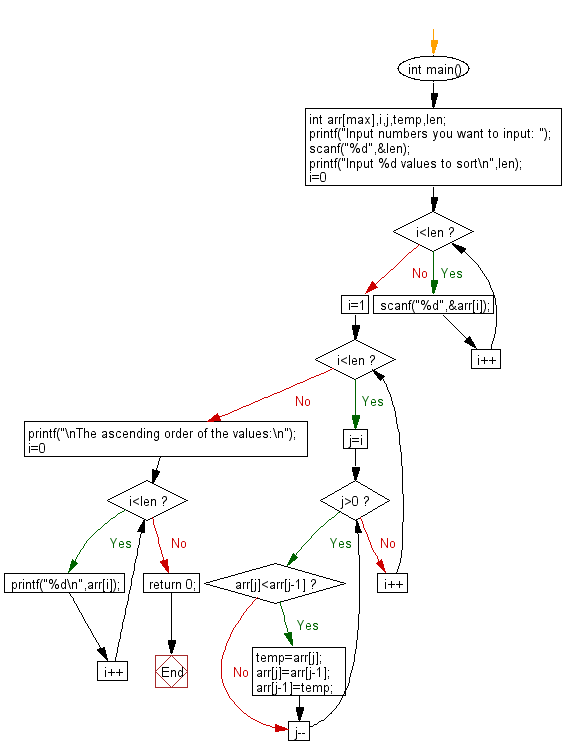﻿ C Program: Returns an array of maximum, minimum values

# C Exercises: Find out maximum and minimum of some values using function which will return an array

## C Function : Exercise-12 with Solution

Write a C program to find the maximum and minimum of some values using a function that returns an array.

Sample Solution:

C Code:

``````# include <stdio.h>
# define max 10
int *maxmin(int ar[], int v);
int main()
{
int  arr[max];
int n,i, *p;
printf("Number of values you want to input: ");
scanf("%d",&n);
printf("Input %d values\n", n);
for(i=0;i<n;i++)
scanf("%d",&arr[i]);
p=maxmin(arr,n);
printf("Minimum value is: %d\n",*p++);
printf("Maximum value is: %d\n",*p);
}
int *maxmin(int arra1[], int v)
{
int i;
static int result_mm;
result_mm=arra1;
result_mm=arra1;
for (i=1;i<v;i++)
{
if(result_mm > arra1[i])
result_mm=arra1[i];
if(result_mm< arra1[i])
result_mm= arra1[i];
}
return result_mm;
}
```
```

Sample Output:

``` Number of values you want to input: Input 5 values
Minimum value is: 11
Maximum value is: 65
```

Explanation:

```int * maxmin(int arra1[], int v) {
int i;
static int result_mm;
result_mm = arra1;
result_mm = arra1;
for (i = 1; i < v; i++) {
if (result_mm > arra1[i])
result_mm = arra1[i];
if (result_mm < arra1[i])
result_mm = arra1[i];
}
return result_mm;
}
```

The 'maxmin' function takes two arguments array 'arra1' type integer and an integer 'v', representing the size of the array. The function finds the minimum and maximum elements of the array arra1 by iterating through all elements of the array and keeping track of the minimum and maximum elements seen so far.

The function initializes a static integer array result_mm of size 2 and initializes its first two elements to the first element of the input array arra1. It then enters a for loop that continues from 1 to v-1. In each iteration of the loop, the function checks whether the current element of the array arra1[i] is greater than the current maximum element result_mm. If it is, result_mm is updated to the value of arra1[i].

Similarly, the function checks whether arra1[i] is less than the current minimum element result_mm. If it is, result_mm is updated to the value of arra1[i].

Finally, the loop completes and the function returns the 'result_mm' array containing the minimum and maximum elements of the input array.

Time complexity and space complexity:

The time complexity of this function is O(n), where n is the input size v, because the for loop iterates n-1 times to find the minimum and maximum elements of the array.

The space complexity of this function is O(1), as it only uses a fixed amount of memory to store the integer array result_mm, which has a constant size of 2.

Flowchart:C Programming Code Editor:

What is the difficulty level of this exercise?

Test your Programming skills with w3resource's quiz.

﻿